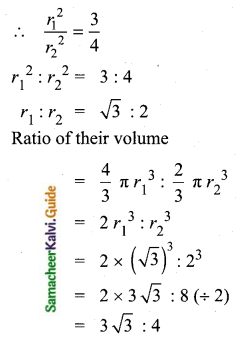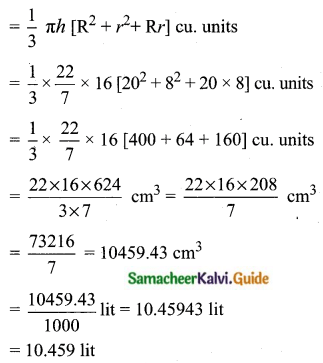Students can download Maths Chapter 7 Mensuration Ex 7.2 Questions and Answers, Notes, Samacheer Kalvi 10th Maths Guide Pdf helps you to revise the complete Tamilnadu State Board New Syllabus, helps students complete homework assignments and to score high marks in board exams.

## Tamilnadu Samacheer Kalvi 10th Maths Solutions Chapter 7 Mensuration Ex 7.2

Question 1.
A 14 m deep well with inner diameter 10 m is dug and the earth taken out is evenly spread all around the well to form an embankment of width 5 m. Find the height of the embankment.Radius of the well (r1) = 5 m
Depth of the well (h) = 14 m
Width of the embankment = 5 m
Outer radius (R) = 5 + 5 = 10 m
Let the height of the embankment be “H”
Volume of Earth in the embankment = Volume of the well
πH(R2 – r2) = $$\pi r_{1}^{2} h$$
H(102 – 52) = 5 × 5 × 14
H (100 – 25) = 5 × 5 × 14
H = $$\frac{5 \times 5 \times 14}{75}$$ = 4.67 m
Height of the embankment = 4.67 mQuestion 2.
A cylindrical glass with diameter 20 cm has water to a height of 9 cm. A small cylindrical metal of radius 5 cm and height 4 cm is immersed it completely. Calculate the rise of the water in the glass?
Radius of the cylindrical glass (r) = 10 cm
Height of the water (h) = 9 cm
Radius of the cylindrical metal (R) = 5 cm
Height of the metal (H) = 4 cm
Let the height of the water raised be “h”
Volume of the water raised in the cylinder = Volume of the cylindrical metal
πr2h = πr2H
10 × 10 × h = 5 × 5 × 4
h = $$\frac{5 \times 5 \times 4}{10 \times 10}$$ = 1 cm
Raise of water in the glass = 1 cmQuestion 3.
If the circumference of a conical wooden piece is 484 cm then find its volume when its height is 105 cm.
Circumference of the wooden piece = 484 cm
2πr = 484
2 × $$\frac{22}{7}$$ × r = 484 cm
r = $$\frac{484 \times 7}{2 \times 22}$$
r = 77 cm
Height of the wooden piece (h) = 105 cm
Volume of the conical wooden piece = $$\frac{1}{3} \pi r^{2} h$$ cu.units
= $$\frac{1}{3} \times \frac{22}{7} \times 77 \times 77 \times 105 \mathrm{cm}^{3}$$
= 22 × 11 × 77 × 35 cm3
= 652190 cm3
Volume of the wooden piece = 652190 cm3Question 4.
A conical container is fully filled with petrol. The radius is 10m and the height is 15 m. If the container can release the petrol through its bottom at the rate of 25 cu. meter per minute, in how many minutes the container will be emptied. Round off your answer to the nearest minute.
The radius of the conical container (r) = 10 m
Height of the container (h) = 15 mQuestion 5.
A right-angled triangle whose sides are 6 cm, 8 cm and 10 cm is revolved about the sides containing the right angle in two ways. Find the difference in volumes of the two solids so formed.Three sides of a triangle are 6 cm, 8 cm and 10 cm.
Case (i): If the triangle is revolved about the side 6 cm, the cone will be formed with radius 6 cm and height 8 cm.
Volume of the cone = $$\frac{1}{3} \pi r^{2} h$$ cu. units
= $$\frac{1}{3}$$ × π × 6 × 6 × 8 = 96π cm3
Case (ii): If the triangle is revolved about the side 8 cm, the cone will be formed with radius 8 cm and height 6 cm.
Volume of the cone = $$\frac{1}{3}$$ × π × 8 × 8 × 6 = 128π cm3
Difference in volume of the two solids = (128π – 96π) cm3 = 32π cm3 = 32 × $$\frac{22}{7}$$ cm3 = 100.57 cm3
The difference in the volume of the two solids = 100.57 cm3

Question 6.
The volumes of two cones of same base radius are 3600 cm3 and 5040 cm3. Find the ratio of heights.
Let the radius of the two cones be ‘r’
Let the height of the two cones be h1 and h2
Ratio of their volumes = 3600 : 5040 (÷ 10)
$$\frac{1}{3} \pi r^{2} h_{1}: \frac{1}{3} \pi r^{2} h_{2}$$ = 360 : 504 (÷4)
h1 : h2 = 90 : 126 (÷3)
= 30 : 42 (÷3)
= 10 : 14 (÷2)
h1 : h2 = 5 : 7
Ratio of heights = 5 : 7Question 7.
If the ratio of radii of two spheres is 4 : 7, find the ratio of their volumes.
Let the ratio of their radii is r1 : r2
r1 : r2 = 4 : 7
Ratio of their volumes
V1 : V2 = $$\frac{4}{3} \pi r_{1}^{3}: \frac{4}{3} \pi r_{2}^{3}$$
= $$r_{1}^{3}: r_{2}^{3}$$
= 43 : 73
Ratio of their volumes = 64 : 343

Question 8.
A solid sphere and a solid hemisphere have an equal total surface area. Prove that the ratio of their volume is 3√3 : 4.
Total surface area of a sphere = $$4 \pi r_{1}^{2}$$ sq. units
Total surface area of a hemisphere = $$3 \pi r_{2}^{2}$$ sq. units
Ratio of Total surface area = $$4 \pi r_{1}^{2}: 3 \pi r_{2}^{2}$$
1 = $$\frac{4 \pi r_{1}^{2}}{3 \pi r_{2}^{2}}$$ (Same Surface Area)
1 = $$\frac{4 r_{1}^{2}}{3 r_{2}^{2}}$$Ratio of their volumes = 3√3 : 4
Hence it is proved.Question 9.
The outer and the inner surface areas of a spherical copper shell are 576π cm2 and 324π cm2 respectively. Find the volume of the material required to make the shell.
Outer surface area of a spherical shell = 576π cm2
4πR2 = 576π
4 × R2 = 576
R2 = $$\frac{576}{4}$$ = 144
R = √144 = 12 cm
Inner surface area of a spherical shell = 324π cm2
4πr2 = 324π
4r2 = 324
r2 = 81
r = √81 = 9
Volume of the material required = Volume of the hollow hemisphere = $$\frac{4}{3}$$ π(R3 – r3) cm3Volume of the material required = 4186.29 cm3

Question 10.
A container open at the top is in the form of a frustum of a cone of height 16 cm with radii of its lower and upper ends are 8 cm and 20 cm respectively. Find the cost of milk which can completely fill a container at the rate of ₹ 40 per litre.